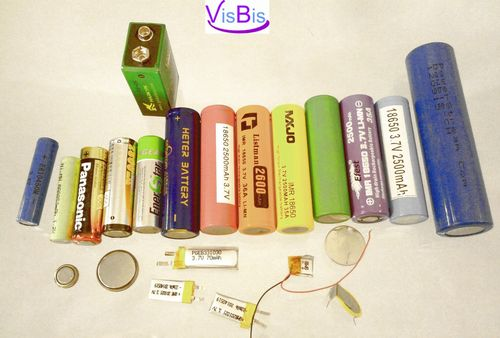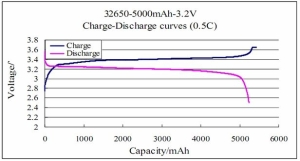# Batteries, basics about batteries, Part!: Introduction and Vocabulary/Semantics

At this point in time battery world experiencing revolution in shapes configurations and chemistry. Sometimes it is confusing to understand which battery is the best to use for your particulate application. Also users sometimes can’t find the right substitution for the battery they had before. I will try to help in this article to understand what is what and basic ideas about batteries.The most common are carbon-zinc, alkaline, lead acid, nickle metal hydride, nickle cadmium and lithium ion. But there are many other types of chemistry, which used more seldom. Below we discuss different of the battery designs currently used, some of the chemistry involved, and advantages and disadvantages of each design. We have also included some useful definitions and a list of parameters to guide you in matching your battery requirements to a specific battery design.

________________________________________
Definitions
Anode: The electrode where oxidation (loss of electrons) takes place. While discharging, it is the negative electrode; while charging it becomes the positive electrode.
• Amps (A): Also known as Amperes. This is the rate at which electrons flow in a wire. Or simply “Current”
• Amp hours (Ahr, mAh, mah etc): Also known as ampere hours. This is a measure of the amount of charge stored or used. This parameter characterize how “big” is the battery in electrical sense.
So Amp-Hours, (AH), or milliamp-Hours (mAH) is a measure of the size of the battery a 200 mAH battery is twice as big as a 100 mAH battery, even though they may be in the same physical dimension and size.
• Batteries: Two or more electrochemical cells, electrically interconnected,
• Battery cell: One battery of given chemistry, not connected in formation. Normally it contains two electrodes and an electrolyte. The redox (oxidation-reduction) reactions that occur at these electrodes convert electrochemical energy into electrical energy. Very often people commonly calling something a “battery” but intermixing the definitions of a single sell or battery pack formed with single cells. Very often it is not obvious that battery does consist of a few single cells.
• C:C represents the capacity of a battery divided by 1 hour, its units are amps. In most of specifications you will commonly find that C parameter. Don’t be confused, it is just the battery capacity in mAh or Ah said in one letter C.
It represents a 1 hour discharge rate using the nominal capacity of the battery. So a discharge rate of 10C for a 5AH battery would be 50 amps. The concept of “C” is also used for charge currents, since both charge and discharge properties are proportional to the capacity of the battery, so a 5C charge rate for a 5 AH battery would be 25 amps.
• Capacity: The total quantity of electricity or total ampere-hours available from a fully charged cell or battery.
• Cathode: The electrode where reduction (gain of electrons) takes place. When discharging, it is the positive electrode, when charging, it becomes the negative electrode.
• Charge: The conversion of electrical energy, provided in the form of current from an external source, into chemical energy stored at the electrodes of a cell or battery.
• Discharge: The conversion of the chemical energy of a cell into electrical energy, which can then be used to supply power to a system.
• Discharge curve: A plot of cell voltage over time into the discharge, at a constant temperature and constant current discharge rate.

Charge-Discharge curve for LiFePO4 batteryTypical discharge curves for LiFePO4 battery

Each curve in this graph represents cell performance at a different discharge rate. The farther right the curve ends, the lower the discharge rate.
• Dry cell: A Leclanché cell, so called because of its non-fluid electrolyte (to prevent spillage). This is achieved by adding an inert metal oxide so that the electrolyte forms a gel or paste.
• Efficiency: For a secondary cell, the ratio of the output on discharge to the input required to restore it to its initial state of charge under specified conditions. Can be measured in ampere-hour, voltage, and watt-hour efficiency.
• Electrolyte: The chemistry of a battery requires a medium that provides the ion transport mechanism between the positive and negative electrodes of a cell.
• Energy density (specific energy): These two terms are often used interchangeably. Energy density refers mainly to the ratio of a battery’s available energy to its volume (watt hour/liter). Specific energy refers to the ratio of energy to mass (watt hour/kg). The energy is determined by the charge that can be stored and the cell voltage (E=qV).
• Fuel cell: A cell in which one or both of the reactants are used in the battery to convert it to electricity. Unlike the metal anodes typically used in batteries, the fuels in a fuel cell are usually gas or liquid, with oxygen as the oxidant. The hydrogen/oxygen fuel cell is the most common. In this fuel cell, hydrogen is oxidized at the anode: 2H2 > 4H+ + 4e

•Polarization: The voltage drop in a cell during discharge due to the flow of an electrical current. The cell’s internal resistance increases with the buildup of a product of oxidation or a reduction of an electrode, preventing further reaction.

•Power: Measured by Watts. Power: P=VI. where V is voltage (V) and I is current ,

Since V=IR, P=I2R and P=V2/R

Power also can be described by energy emitted per unit of time: P=E/t.

Thus E=VIt=qV.

•Power density (specific power): Power density is the ratio of the power available from a battery to its volume (watts/liter). Specific power generally refers to the ratio of power to mass (watts/kg). Comparison of power to cell mass is more common.

•Primary cells: A cell that is not designed for recharging and is discarded once it has produced all its electrical energy.

•Prismatic: Just a word to say that the cells are not cylindrical, as nature intended battery cells to be, but fit nicely into a parallelepiped, rectangular or any other such flattened shape.

Viewed: 883